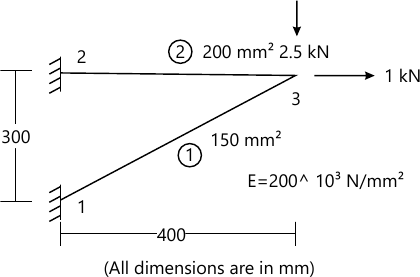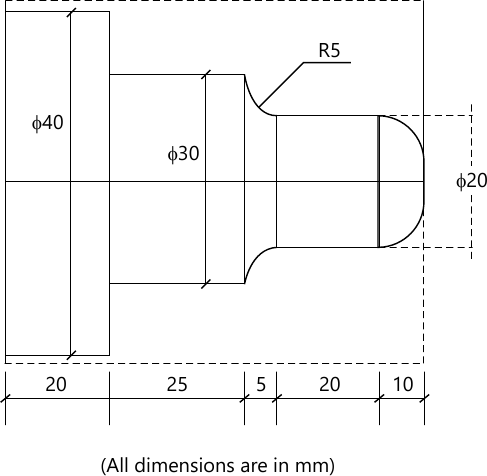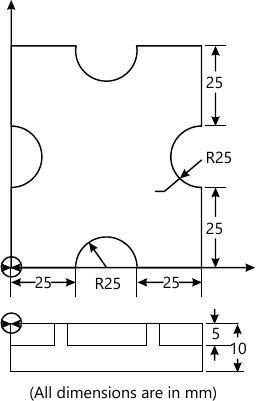SPPU Mechanical Engineering (Semester 7)
December 2015
Total marks: --
Total time: --
INSTRUCTIONS
(1) Assume appropriate data and state your reasons
(2) Marks are given to the right of every question
(3) Draw neat diagrams wherever necessary

Solve any one question from Q1 and Q2
1 (a) Line A(5, 5) B(10, 15) is to be rotated about point B by 60° in CCW direct find the new position of point A and B of line.
6 M
1 (b) In concatenated transformation why translation matrix to be written in homogeneous form also write translation matrix in homogeneous form.
4 M

2 (a) Explain Geometric translational and rotational mapping and its need.
6 M
2 (b) Explain Bezier synthetic curve.
4 M

Solve any one question from Q3 and Q4
3 (a) Explain CSG technique of solid modelling and its advantages and limitation.
6 M
3 (b) Explain the concept of shape function for 1-D element.
4 M

4 For the truss element shown in the figure find Global stiffness matrix and write in the form KQ=F. Compute nodal displacements.10 M

Solve any one question from Q5 and Q6
5 (a) Explain the canned cycle for Drilling, Boring and Tapping.
8 M
5 (b) Write CNC part program for roughing and finishing using canned cycle for turned component as shown in figure. Assume Suitable cutting data.10 M

6 (a) Explain the concept of tool radius compensation while CNC programming and explain G codes and its format for cutter compensation.
6 M
6 (b) Write CNC part program for Milling and Drilling for component as shown in figure use concept of sub programming so that depth of cut per pass is 1 mm. Assume Suitable cutting data.12 M

Solve any one question from Q7 and Q8
7 (a) Explain Rapid Prototyping (RP) systems in detail. List various RP techniques. Explain 3-D Printing with neat sketch.
8 M
7 (b) Explain Rapid Tooling in detail. List different Rapid Tooling methods. State benefits of them.
8 M

8 (a) Write steps in Fused Deposition Modelling (FDM) with neat sketch. State its applications.
8 M
8 (b) Write steps in Selective Laser Sintering method with neat sketch. State its advantages.
8 M

Solve any one question from Q9 and Q10
9 (a) Classify robot on the basis of configuration and explain Articulated configuration of Robot with advantages limitation and applications.
10 M
9 (b) Explain various joints used in Robot.
6 M

10 (a) Explain the FMS system with its relevance in present era of Flexible Automation.
8 M
10 (b) Explain Group Technology. Explain the optiz coding system.
8 M

More question papers from CAD/CAM Automation